# Heat Energy Internal energy Kinetic Energy Potential Energy

• Slides: 17Heat Energy Internal energy Kinetic Energy Potential Energy Endothermic Exothermic Thermodynamics Thermal Equilibrium System Surroundings Law of Conservation of Energy Heat Capacity Specific Heat Capacity First Law of Thermodynamics Melting Freezing Deposition Sublimation Evaporation Condensation State Function Standard state temperature Standard state pressure Standard states matter Enthalpy Hess’s Law Thermochemical Equation Enthalpy of Formation Intramolecular forces Intermolecular forces Hydrogen Bonding Polarization Polarizability Vapor Pressure Equilibrium Heat of Vaporization Phase Diagram Solid Liquid Gas Triple Point Critical Point Super Critical FluidIsomers structural isomers constitutional isomers stereo isomers racemic mixture entantiomers geometric isomers positional isomers chiral molecules chiral centers optical isomers cis mer trans fac hydration isomers ionization isomers coordination isomers linkage isomers titration titrant primary standard secondary standard end point equivalence point p. H oxidation numbers hydrocarbons unsaturated hydrocarbons alkanes alkenes alkynes aromatic compounds alkyls phenols alcohols esters ethers carbonyl groups aldehydes ketones carboxylic acids acyl chlorides organic halides amines amides resonance Arrhenius acids/bases Brönsted/Lowery acids/bases Lewis acids/bases sugars polymers solvent concentration ppm wt% molecular equations ionic equations net ionic equations spectator ion metathesis reaction combination reaction decomposition reaction displacement reaction redox reaction addition polymerization condensation polymerization ligand donor atom unidentate polydentate chelate coordination number coordination sphere fats solution solute molarity ppb vol%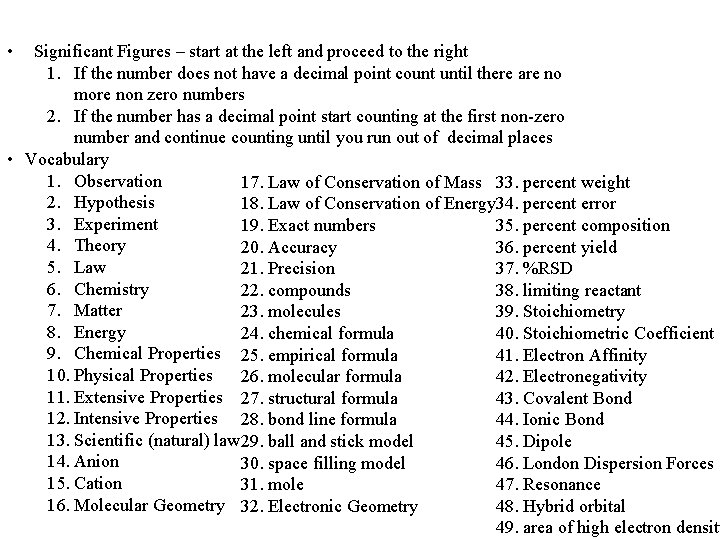• Significant Figures – start at the left and proceed to the right 1. If the number does not have a decimal point count until there are no more non zero numbers 2. If the number has a decimal point start counting at the first non-zero number and continue counting until you run out of decimal places • Vocabulary 1. Observation 17. Law of Conservation of Mass 33. percent weight 2. Hypothesis 18. Law of Conservation of Energy 34. percent error 3. Experiment 19. Exact numbers 35. percent composition 4. Theory 20. Accuracy 36. percent yield 5. Law 21. Precision 37. %RSD 6. Chemistry 22. compounds 38. limiting reactant 7. Matter 23. molecules 39. Stoichiometry 8. Energy 24. chemical formula 40. Stoichiometric Coefficient 9. Chemical Properties 25. empirical formula 41. Electron Affinity 10. Physical Properties 26. molecular formula 42. Electronegativity 11. Extensive Properties 27. structural formula 43. Covalent Bond 12. Intensive Properties 28. bond line formula 44. Ionic Bond 13. Scientific (natural) law 29. ball and stick model 45. Dipole 14. Anion 30. space filling model 46. London Dispersion Forces 15. Cation 31. mole 47. Resonance 16. Molecular Geometry 32. Electronic Geometry 48. Hybrid orbital 49. area of high electron density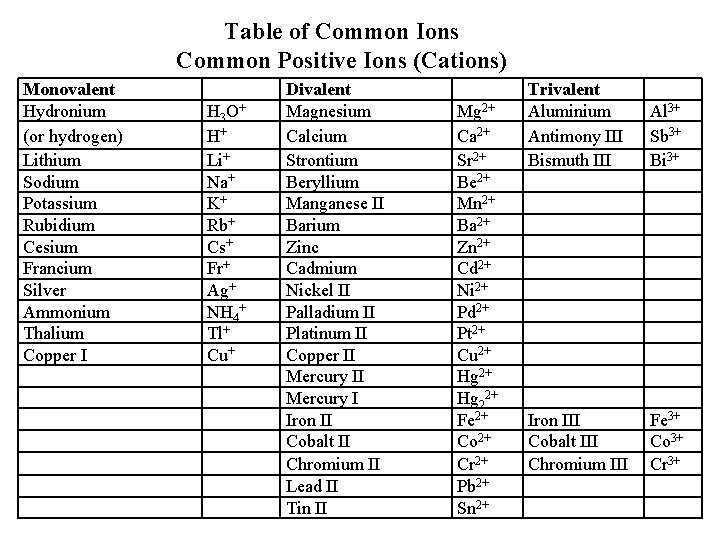Table of Common Ions Common Positive Ions (Cations) Monovalent Hydronium (or hydrogen) Lithium Sodium Potassium Rubidium Cesium Francium Silver Ammonium Thalium Copper I H 3 O+ H+ Li+ Na+ K+ Rb+ Cs+ Fr+ Ag+ NH 4+ Tl+ Cu+ Divalent Magnesium Calcium Strontium Beryllium Manganese II Barium Zinc Cadmium Nickel II Palladium II Platinum II Copper II Mercury I Iron II Cobalt II Chromium II Lead II Tin II Mg 2+ Ca 2+ Sr 2+ Be 2+ Mn 2+ Ba 2+ Zn 2+ Cd 2+ Ni 2+ Pd 2+ Pt 2+ Cu 2+ Hg 22+ Fe 2+ Co 2+ Cr 2+ Pb 2+ Sn 2+ Trivalent Aluminium Antimony III Bismuth III Al 3+ Sb 3+ Bi 3+ Iron III Cobalt III Chromium III Fe 3+ Co 3+ Cr 3+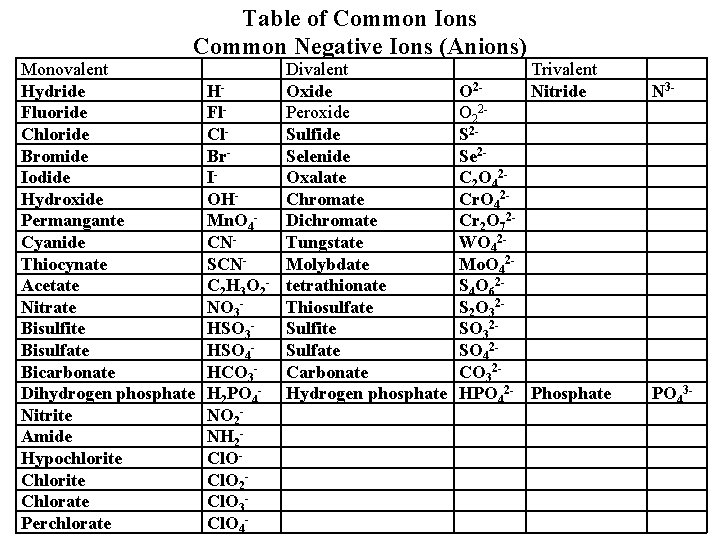Table of Common Ions Common Negative Ions (Anions) Monovalent Hydride Fluoride Chloride Bromide Iodide Hydroxide Permangante Cyanide Thiocynate Acetate Nitrate Bisulfite Bisulfate Bicarbonate Dihydrogen phosphate Nitrite Amide Hypochlorite Chlorate Perchlorate HFl. Cl. Br. IOHMn. O 4 CNSCNC 2 H 3 O 2 NO 3 HSO 4 HCO 3 H 2 PO 4 NO 2 NH 2 Cl. O 3 Cl. O 4 - Divalent Oxide Peroxide Sulfide Selenide Oxalate Chromate Dichromate Tungstate Molybdate tetrathionate Thiosulfate Sulfite Sulfate Carbonate Hydrogen phosphate Trivalent Nitride O 2 O 22 S 2 Se 2 C 2 O 42 Cr 2 O 72 WO 42 Mo. O 42 S 4 O 62 S 2 O 32 SO 42 CO 32 HPO 42 - Phosphate N 3 - PO 43 -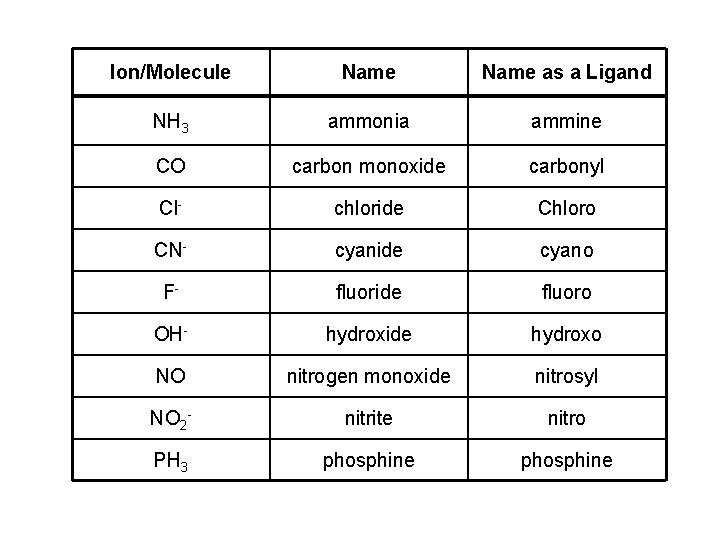Ion/Molecule Name as a Ligand NH 3 ammonia ammine CO carbon monoxide carbonyl Cl- chloride Chloro CN- cyanide cyano F- fluoride fluoro OH- hydroxide hydroxo NO nitrogen monoxide nitrosyl NO 2 - nitrite nitro PH 3 phosphine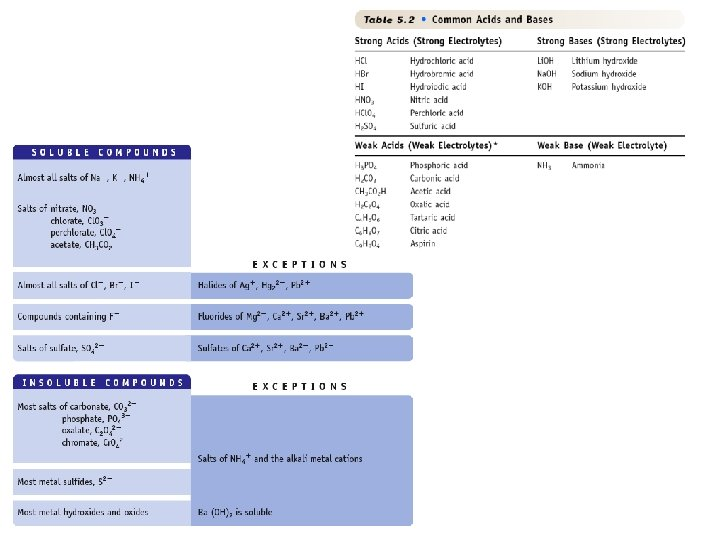Standard P 1. 00000 atm or 101. 3 k. Pa Standard T 273. 15 K or 0. 00 o. C K = 273 + o. C 1 mm Hg = 1 torr 760 torr = 1 atm The standard molar volume is 22. 4 L at STP Variable Cond. 1 Cond. 2 0. 08206 P (atm) PV = n. RT R = 0. 08206 L atm V (L) mol-1 K-1 Ptotal = PA + PB + PC +. . . N (moles) R (L atm mol-1 K-1) T (K) At low temperatures and high pressures real gases do not behave ideally. The reasons for the deviations from ideality are: 1. The molecules are very close to one another, thus their volume is important. 2. The molecular interactions also become important.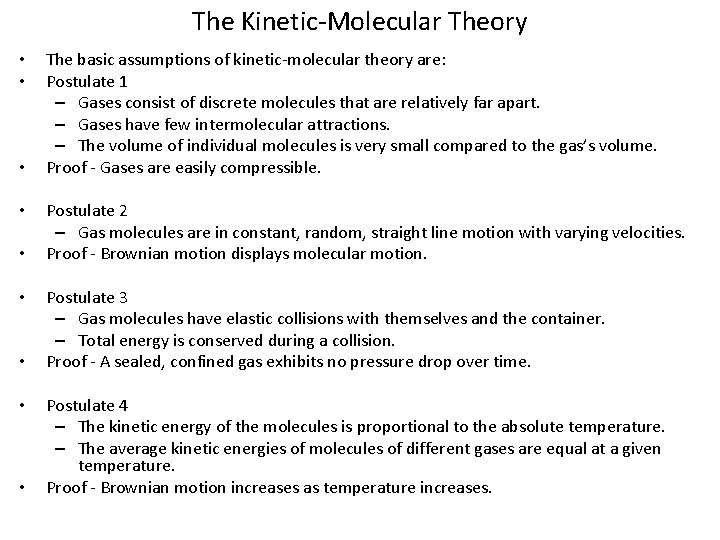The Kinetic-Molecular Theory • • • The basic assumptions of kinetic-molecular theory are: Postulate 1 – Gases consist of discrete molecules that are relatively far apart. – Gases have few intermolecular attractions. – The volume of individual molecules is very small compared to the gas’s volume. Proof - Gases are easily compressible. Postulate 2 – Gas molecules are in constant, random, straight line motion with varying velocities. Proof - Brownian motion displays molecular motion. Postulate 3 – Gas molecules have elastic collisions with themselves and the container. – Total energy is conserved during a collision. Proof - A sealed, confined gas exhibits no pressure drop over time. Postulate 4 – The kinetic energy of the molecules is proportional to the absolute temperature. – The average kinetic energies of molecules of different gases are equal at a given temperature. Proof - Brownian motion increases as temperature increases.∆H = Hfinal - Hinitial • The stoichiometric coefficients in thermochemical equations must be interpreted as numbers of moles. 1 mol of C 5 H 12 reacts with 8 mol of O 2 to produce 5 mol of CO 2, 6 mol of H 2 O, and releasing 3523 k. J is referred to as one mole of reactions. ∆Horxn = ∆Hfo (prod) - ∆Hfo (react) Specific heat capacity (J/(g∙K) = c. P = q m(Tf –Ti) heat lost or gained by system (Joules) mass(grams) DT (Kelvins) Variable System 1 Cp Tf Ti m q System 2heat transfer in (endothermic), +q heat transfer out (exothermic), -q SYSTEM ∆E = q + w w transfer in (+w) w transfer out (-w)Carbon Atom Hybridization C uses C forms Example sp 3 tetrahedral 4 sp 3 hybrids 4 bonds CH 4 sp 2 trigonal planar 3 sp 2 hybrids & 1 p orbital 3 bonds 1 bond C 2 H 4 sp linear 2 sp hybrids & 2 p orbitals 2 bonds C 2 H 2organic nomenclature and structure inorganic nomenclature and structure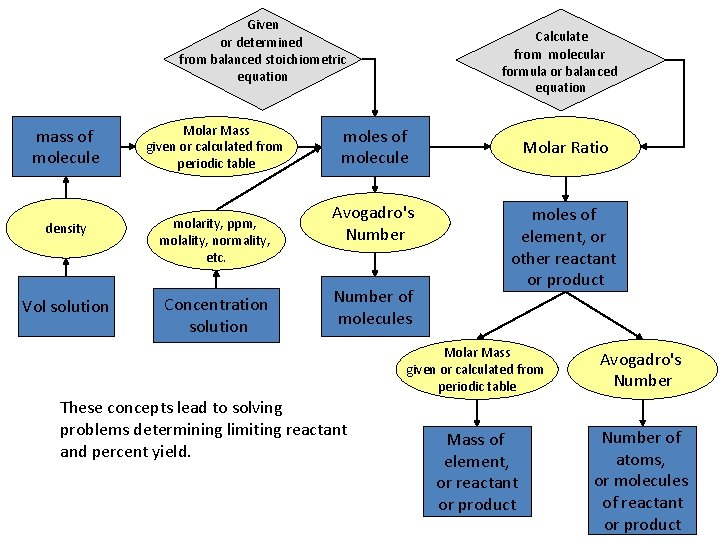Given or determined from balanced stoichiometric equation mass of molecule Molar Mass given or calculated from periodic table density molarity, ppm, molality, normality, etc. Vol solution Concentration solution Calculate from molecular formula or balanced equation moles of molecule Avogadro's Number of molecules These concepts lead to solving problems determining limiting reactant and percent yield. Molar Ratio moles of element, or other reactant or product Molar Mass given or calculated from periodic table Avogadro's Number Mass of element, or reactant or product Number of atoms, or molecules of reactant or productQuantum Numbers n and l define the energy of the electron The principal quantum number has the symbol ~ n which defines the energy of the shell n = 1, 2, 3, 4, . . . “shells” The angular momentum quantum number has the symbol ~ which defines the subshells. = 0, 1, 2, 3, 4, 5, . . . . (n-1) = s, p, d, f, g, h, . . . . (n-1) The symbol for the magnetic quantum number is m which defines the orbital. m = - , (- + 1), (- +2), . . . 0, . . . . , ( -2), ( -1), The last quantum number is the spin quantum number which has the symbol ms which characterizes the single electron. The one spin up ↑ and one spin down�↓ spin quantum number only has two possible values. ms = +½ or -½ The Nucleus: Electrons: Build by adding the required number of protons Hund’s Rule states that each orbital will be filled singly (the atomic number) and neutrons (the mass of the atom) before pairing begins. The singly filled orbitals will have a parallel spin. Pauli’s Exclusion Principle states that paired electrons in an orbital will have opposite spins. Fill the electrons in starting with the lowest energy level adhering to Hund’s and Pauli’s rules.Ionic Polar Covalent Determine Inductive effect Count the number of electrons the element should have Determine how equally electrons are shared (DEN) >1. 7 consider it ionic Oxidation number Formal charge Never Have a Full Octet Always Have a Full Octet Sometimes Exceed a Full Octet To calculate a formal charge 1. draw the Lewis dot structure 2. draw circles around each atom and the electrons associated with it. Remember that formal charges are associated with covalent bonds and that all electrons are shared equally. 3. compare to the group number for that atom. If the number is larger the formal charge is negative, smaller the formal charge is positive. To calculate an oxidation number 1. list all the elements follow with an equal sign 2. follow with the number of atoms of that type in the molecule 1. follow with a multiplication sign 2. If the element is O follow with a -2 3. If the element is H follow with a +1 4. any other element enter a ? 5. follow with an = sign, do the math 6. draw a total line, then enter the charge on the molecule 7. Do the algebra backwards to solve for ?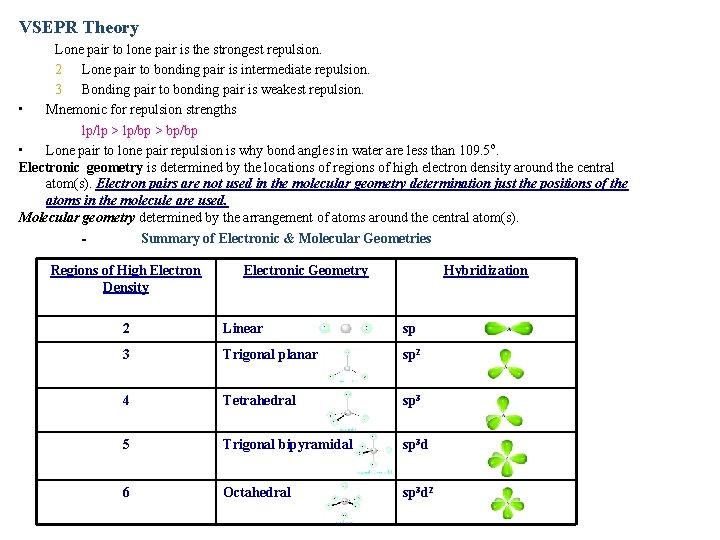VSEPR Theory Lone pair to lone pair is the strongest repulsion. 2 Lone pair to bonding pair is intermediate repulsion. 3 Bonding pair to bonding pair is weakest repulsion. • Mnemonic for repulsion strengths lp/lp > lp/bp > bp/bp • Lone pair to lone pair repulsion is why bond angles in water are less than 109. 5 o. Electronic geometry is determined by the locations of regions of high electron density around the central atom(s). Electron pairs are not used in the molecular geometry determination just the positions of the atoms in the molecule are used. Molecular geometry determined by the arrangement of atoms around the central atom(s). Summary of Electronic & Molecular Geometries Regions of High Electron Density Electronic Geometry Hybridization 2 Linear sp 3 Trigonal planar sp 2 4 Tetrahedral sp 3 5 Trigonal bipyramidal sp 3 d 6 Octahedral sp 3 d 2